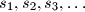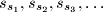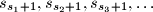### IMO Shortlist 2009 problem A6

Kvaliteta:
Avg: 4,0
Težina:
Avg: 7,5
Suppose that$s_1,s_2,s_3, \ldots$ is a strictly increasing sequence of positive integers such that the sub-sequences$s_{s_1},s_{s_2},s_{s_3},\ldots$ and$s_{s_1 + 1},s_{s_2 + 1},s_{s_3 + 1},\ldots$ are both arithmetic progressions. Prove that the sequence$s_1,s_2,s_3, \ldots$ is itself an arithmetic progression.

Proposed by Gabriel Carroll, USA
Izvor: Međunarodna matematička olimpijada, shortlist 2009